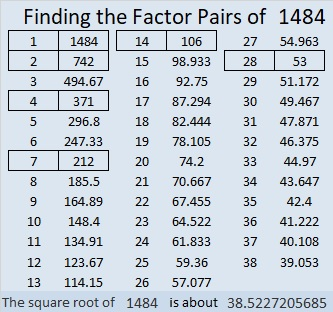# 1484 A Popsicle for a Hot Summer Day

### Today’s Puzzle:

It can be tricky to eat a melting popsicle on a hot summer day. Likewise, it can be tricky to solve a level 6 puzzle even if it looks like a popsicle!  Making it was my granddaughter’s idea. We hope you enjoy it!### Factors of 1484:

If you know that 7 x 12 is 84, it isn’t hard to recognize that 1484 is divisible by 7.

• 1484 is a composite number.
• Prime factorization: 1484 = 2 × 2 × 7 × 53, which can be written 1484 = 2² × 7 × 53
• 1484 has at least one exponent greater than 1 in its prime factorization so √1484 can be simplified. Taking the factor pair from the factor pair table below with the largest square number factor, we get √1484 = (√4)(√371) = 2√371
• The exponents in the prime factorization are 2, 1, and 1. Adding one to each exponent and multiplying we get (2 + 1)(1 + 1)(1 + 1) = 3 × 2 × 2 = 12. Therefore 1484 has exactly 12 factors.
• The factors of 1484 are outlined with their factor pair partners in the graphic below.### Other facts about the Number 1484:

1484 is the difference of two squares in two different ways:
372² – 370² = 1484
60² – 46² = 1484

784-1260-1484 which is 28 times (28-45-53)